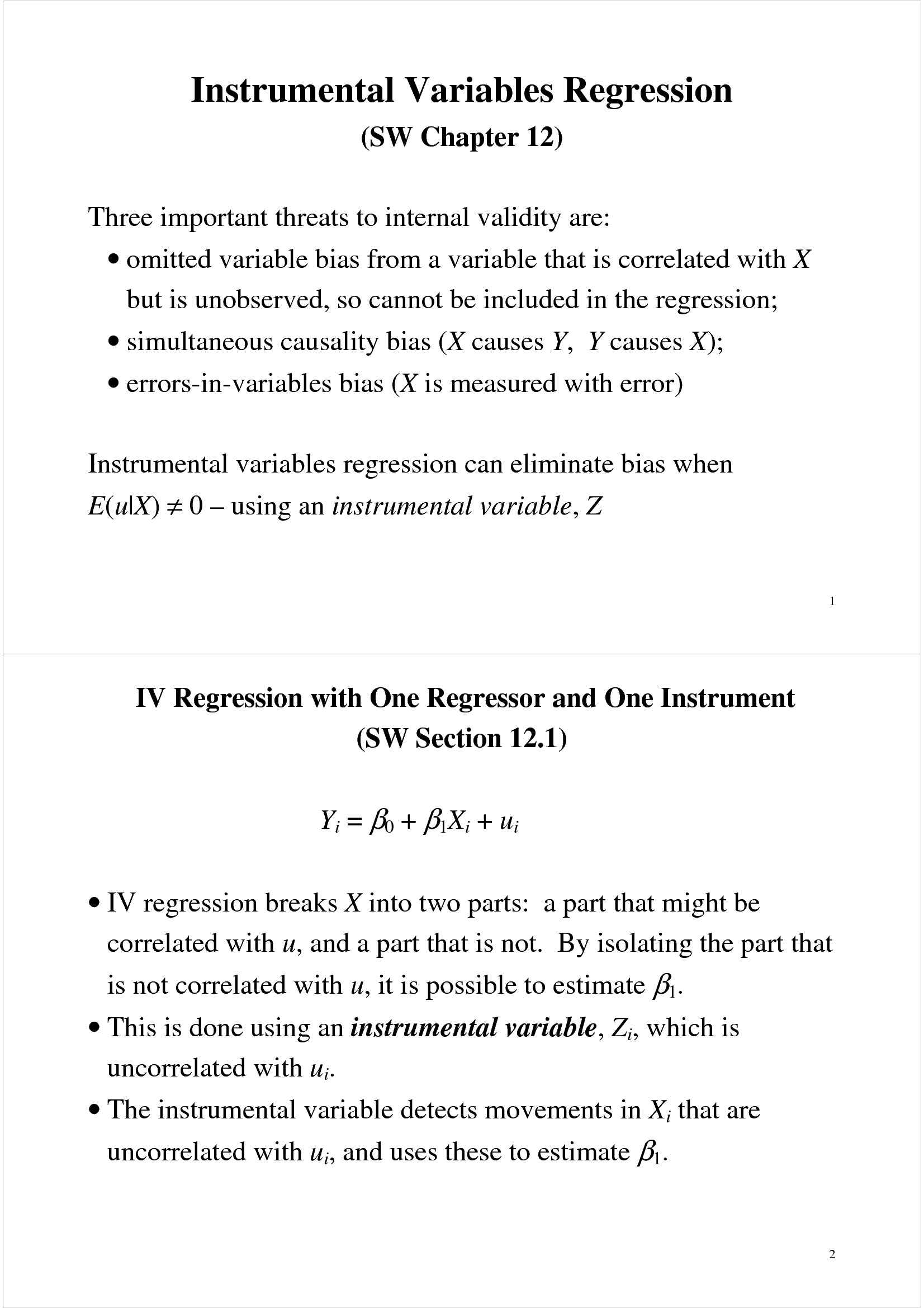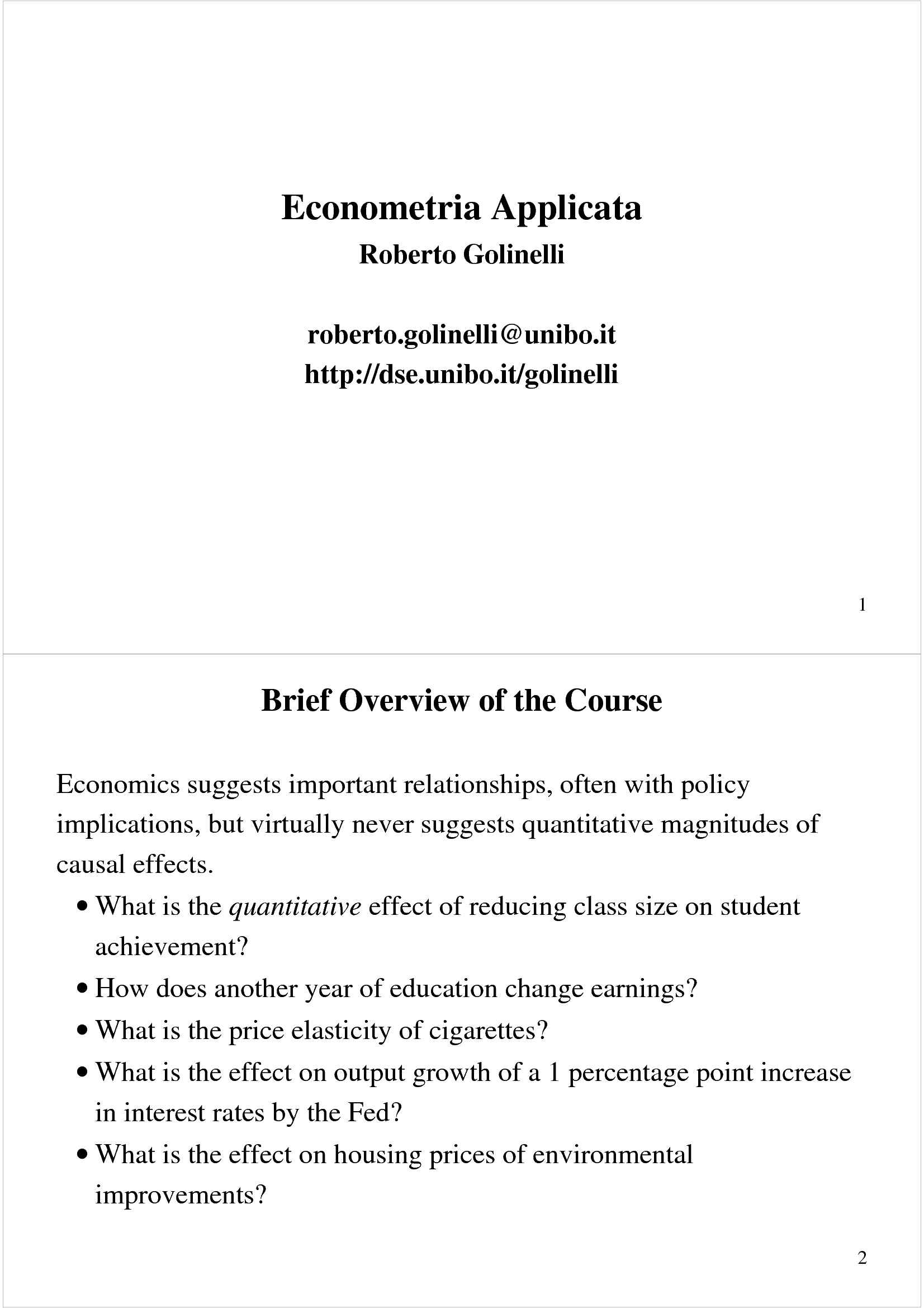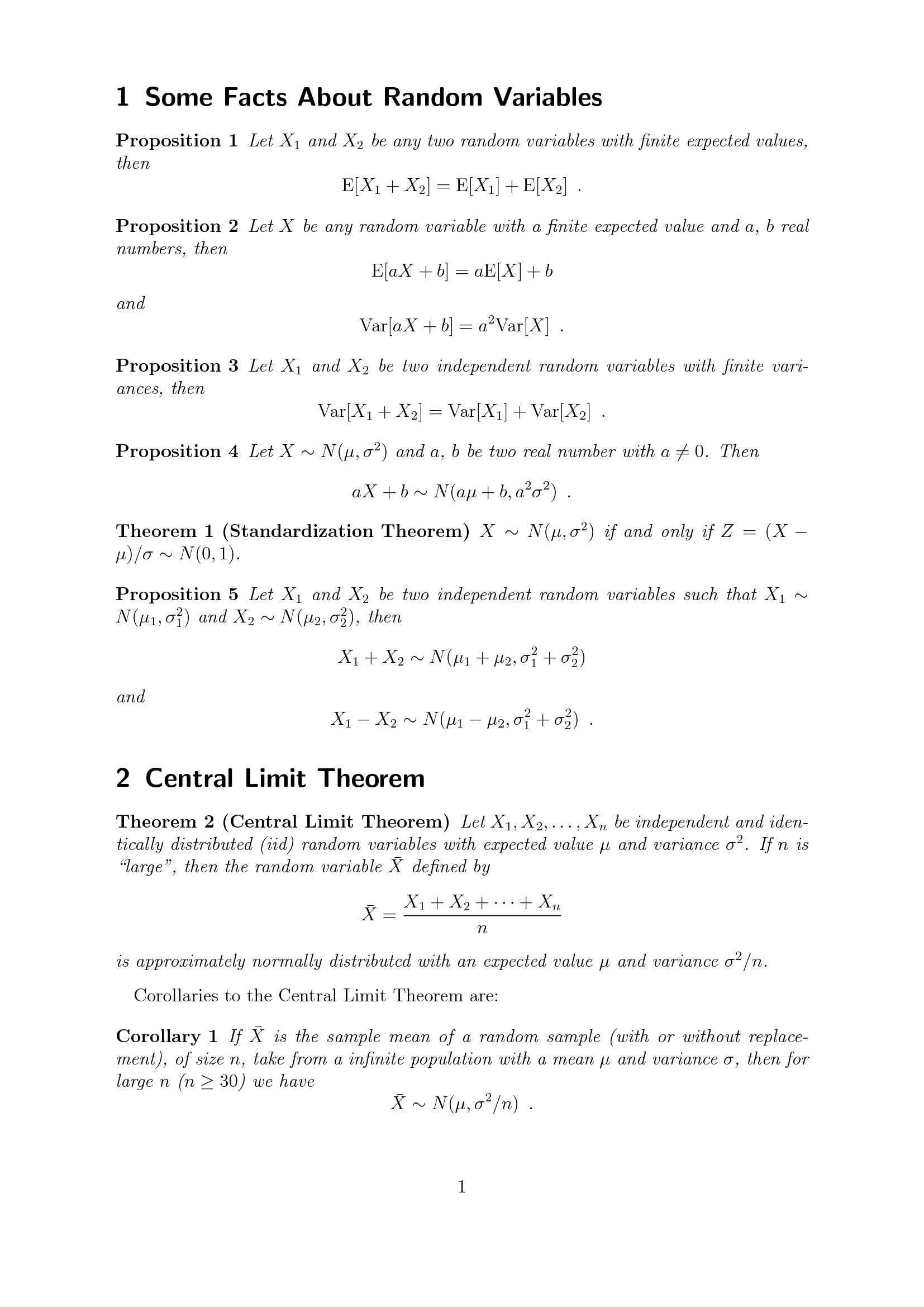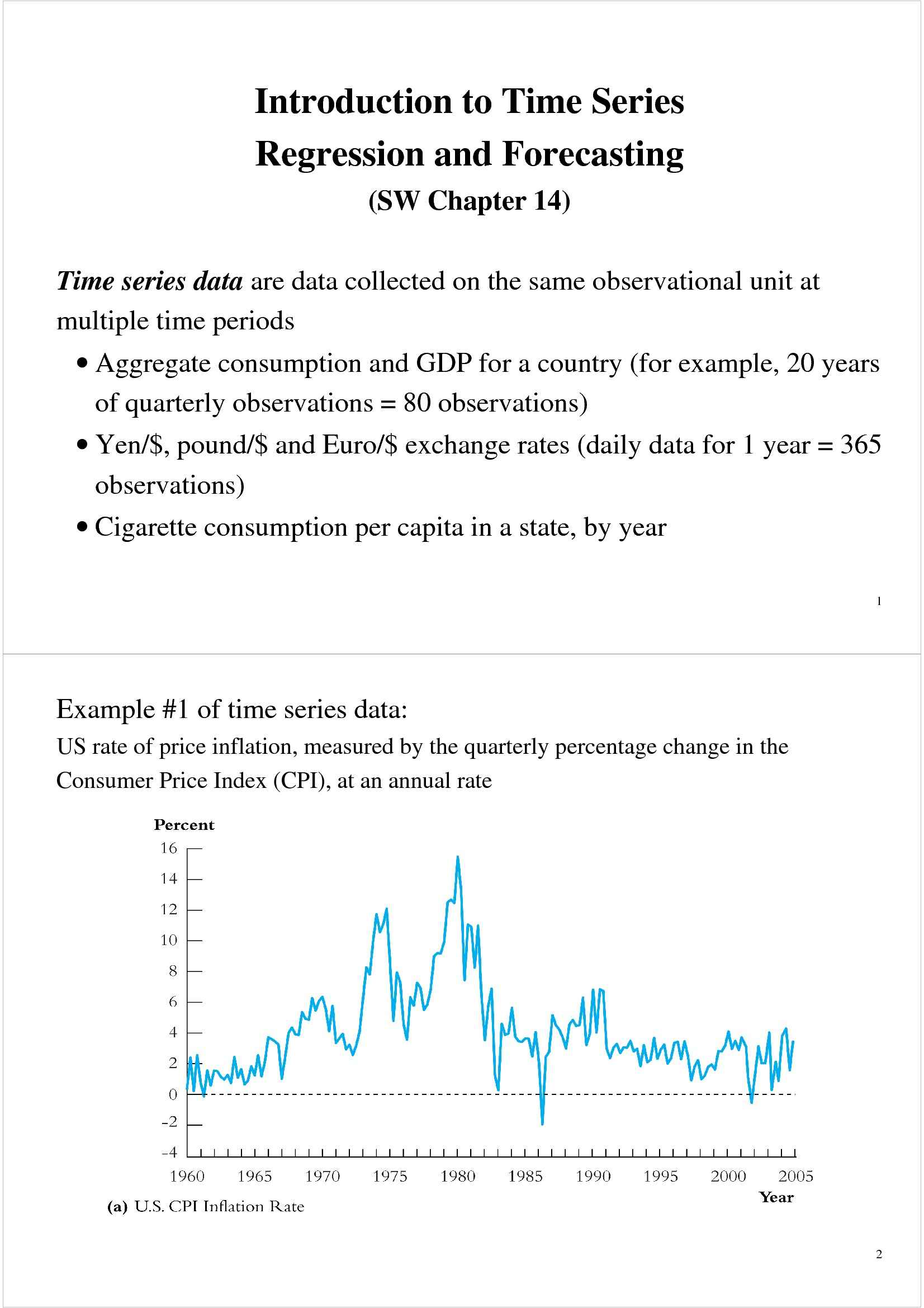Che materia stai cercando?

# Regressione multipla

Materiale didattico per il corso di Econometria applicata del prof. Roberto Golinelli. Trattasi di slides in lingua inglese a cura del docente, all'interno delle quali sono affrontati i seguenti argomenti: introduzione alla regressione multipla; variabili omesse; formula della deviazione della variabile omessa; il... Vedi di più

Esame di Econometria applicata docente Prof. R. Golinelli

Anteprima

### ESTRATTO DOCUMENTO

β β β

Before: Y = + X + X

0 1 1 2 2

β β β

## ∆X

∆Y + (X + ) + X

After: Y + = 0 1 1 1 2 2

β

## ∆Y ∆X

Difference: = 1 1

So: ∆ Y

β = , holding X constant

1 2

∆ X 1

∆ Y

β = , holding X constant

2 1

∆ X 2

β = predicted value of Y when X = X = 0.

0 1 2 13

The OLS Estimator in Multiple Regression

(SW Section 6.3)

With two regressors, the OLS estimator solves:

n

∑ − + + 2

min [

Y ( b b X b X )]

, , 0 1 1 2 2

b b b i i i

0 1 2 =

i 1

• The OLS estimator minimizes the average squared difference between

and the prediction (predicted value) based on

the actual values of Y i

the estimated line.

• This minimization problem is solved using calculus

β β

• This yields the OLS estimators of and .

0 1 14

Example: the California test score data

Regression of testscr against STR:

ˆ

tes t scr = 698.9 – 2.28×STR

Now include percent English Learners in the district (el_pct):

(show the regression on screen)

ˆ

tes t scr = 686.0 – 1.10×STR – 0.65 el_pct

• What happens to the coefficient on STR?

• Why? (Note: corr(STR, el_pct) = 0.19) 15

Measures of Fit for Multiple Regression

(SW Section 6.4) ˆ ˆ

= +

Actual = predicted + residual: Y Y u

i i i

ˆ

SER = std. deviation of (with d.f. correction)

u

i ˆ (without d.f. correction)

RMSE = std. deviation of u

i

2

R = fraction of variance of Y explained by the explanatory X X ... X

1 2 k

2 2

2 = “adjusted R ” = R with a degrees-of-freedom correction

R 2

2

that adjusts for estimation uncertainty; < R

R 16

SER and RMSE

As in regression with a single regressor, the SER and the RMSE are

measures of the spread of the Y’s around the regression line:

n

1 ∑ 2

ˆ

SER = u

− − i

n k 1 =

i 1

n

1 ∑ 2

ˆ

RMSE = u

i

n =

i 1 17

2 2

R and R

2

The R is the fraction of the variance explained – same definition as in

regression with a single regressor:

2 = = ,

R 1

## TSS TSS

n n n

∑ ∑ ∑

− −

ˆ ˆ 2 2 2

ˆ

where ESS = , SSR = , TSS = .

(

Y Y ) u (

Y Y )

i i

i

= = =

i 1 i 1 i 1

• 2

The R always increases when you add another regressor (why?) – a

bit of a problem for a measure of “fit” 18

2 2

R and , ctd.

R 2

2

The (the “adjusted R ”) corrects this problem by “penalizing” you

R 2 does not necessarily increase

for including another regressor – the R

 

n 1 SSR

2 2

: =

R  

− −

 

n k 1 TSS

2

2 < R , however if n is large the two will be very close.

Note that R 19

Measures of fit, ctd.

Test score example:

ˆ

tes t scr = 698.9 – 2.28×STR,

(1) 2 = 0.05, SER = 18.6

## R

ˆ

tes t scr = 686.0 – 1.10×STR – 0.65 el_pct,

(2) 2 2

= 0.426, = 0.424, SER = 14.5

## R R

• What – precisely – does this tell you about the fit of regression (2)

compared with regression (1)?

• 2 2

Why are the R and the so close in (2)?

R 20

The Least Squares Assumptions for Multiple Regression

(SW Section 6.5)

β β β β

= + X + X + … + X + u , i = 1,…,n

Y i 0 1 1i 2 2i k ki i

1. The conditional distribution of u given the X’s has mean zero, that

is, E(u|X = x ,…, X = x ) = 0.

1 1 k k

2. (X ,…,X ,Y ), i =1,…,n, are i.i.d.

1i ki i

3. Large outliers are rare: X ,…, X , and Y have four moments:

1 k

∞,…, ∞, ∞.

4 4 4

E( ) < ( ) < ( ) <

## X E X E Y

1i ki i

4. There is no perfect multicollinearity. 21

Assumption #1: the conditional mean of u given the included X’s is

zero. = x ,…, X = x ) = 0

E(u|X

1 1 k k

• This has the same interpretation as in regression with a single

regressor.

• If an omitted variable (1) belongs in the equation (so is in u) and

(2) is correlated with an included X, then this condition fails

• Failure of this condition leads to omitted variable bias

• The solution – if possible – is to include the omitted variable in the

regression. 22

Assumption #2: (X ,…,X ,Y ), i =1,…,n, are i.i.d.

1i ki i

This is satisfied automatically if the data are collected by simple

random sampling.

Assumption #3: large outliers are rare (finite fourth moments)

This is the same assumption as we had before for a single regressor.

As in the case of a single regressor, OLS can be sensitive to large

outliers, so you need to check your data (scatterplots!) to make sure

there are no crazy values (typos or coding errors).

Assumption #4: There is no perfect multicollinearity

Perfect multicollinearity is when one of the regressors is an exact

linear function of the other regressors. 23

Perfect multicollinearity is when one of the regressors is an exact

linear function of the other regressors. β

• In a regression, if e.g. STR enters twice, is the effect on testscr of a

1

unit change in STR, holding STR constant (???)

with more examples…

With these least squares assumptions in hand, we now can derive the

β β β

ˆ ˆ ˆ

,…, .

sampling distribution of ,

1 2 k 24

The Sampling Distribution of the OLS Estimator

(SW Section 6.6)

Under the four Least Squares Assumptions, β

β β

• ˆ ˆ

has mean , var( ) is

The exact (finite sample) distribution of 1

1 1

β

ˆ .(see

inversely proportional to n; so too for also below)

2

• Other than its mean and variance, the exact (finite-n) distribution of

β

ˆ is very complicated; but for large n…

1 p β

β β

• →

ˆ ˆ (law of large numbers)

is consistent: 1

1 1

β β

ˆ ˆ

E ( )

• 1 1 is approximately distributed N(0,1) (CLT)

β

ˆ

var( )

1 β β

• ˆ ˆ

So too for ,…,

2 k

Conceptually, there is nothing new here! 25

Multicollinearity, Perfect and Imperfect

(SW Section 6.7)

Some more examples of perfect multicollinearity

• The example from earlier: you include STR twice.

• Second example: regress testscr on a constant, D, and B, where: D =

i

= 1 if STR >20, = 0 otherwise, so B =

1 if STR 20, = 0 otherwise; B

≤ i i

1 – D and there is perfect multicollinearity

i

• Would there be perfect multicollinearity if the intercept (constant)

were somehow dropped (that is, omitted or suppressed) in this

regression?

• This example is a special case of… 26

PAGINE

16

PESO

203.04 KB

AUTORE

PUBBLICATO

+1 anno fa

### DESCRIZIONE DISPENSA

Materiale didattico per il corso di Econometria applicata del prof. Roberto Golinelli. Trattasi di slides in lingua inglese a cura del docente, all'interno delle quali sono affrontati i seguenti argomenti: introduzione alla regressione multipla; variabili omesse; formula della deviazione della variabile omessa; il modello di regressione lineare multipla; multicollinearity.

DETTAGLI
Corso di laurea: Corso di laurea in economia, mercati e istituzioni
SSD:
Università: Bologna - Unibo
A.A.: 2011-2012

I contenuti di questa pagina costituiscono rielaborazioni personali del Publisher Atreyu di informazioni apprese con la frequenza delle lezioni di Econometria applicata e studio autonomo di eventuali libri di riferimento in preparazione dell'esame finale o della tesi. Non devono intendersi come materiale ufficiale dell'università Bologna - Unibo o del prof Golinelli Roberto.

Acquista con carta o conto PayPal

Scarica il file tutte le volte che vuoi

Paga con un conto PayPal per usufruire della garanzia Soddisfatto o rimborsato

RecensioniTi è piaciuto questo appunto? Valutalo!

## Altri appunti di Econometria applicataDispensa

### Regressione con variabili strumentaliDispensa

### Econometria - elementiDispensa

### Riepilogo di concetti statisticiDispensa